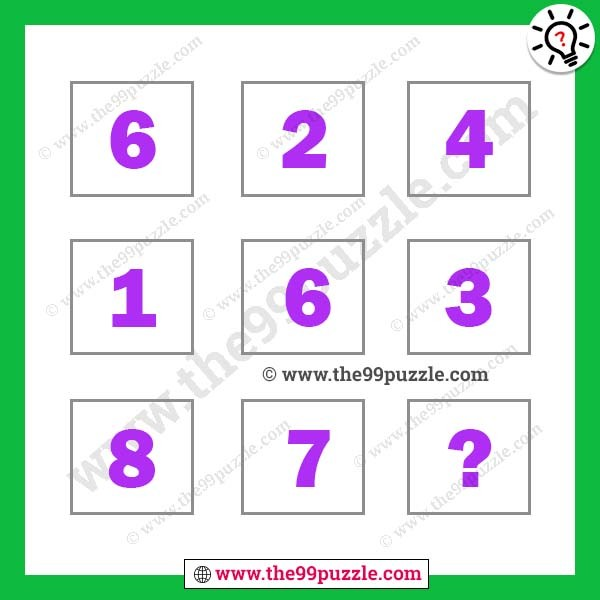# Finding missing number maths puzzle – Puzz169

Finding missing number maths puzzle. Test your mathematical skills as well as logical reasoning skills with the mathematical logical Brain Teaser. In this simple logical number puzzle, there is some logic. can you find the missing number maths puzzle?###### Explanation:

The Sum of each column is equal.

1st Column=6+1+8=15

2nd Column=2+6+7=15

3rd Column=4+3+8=15